##### Differential Equations For Dummies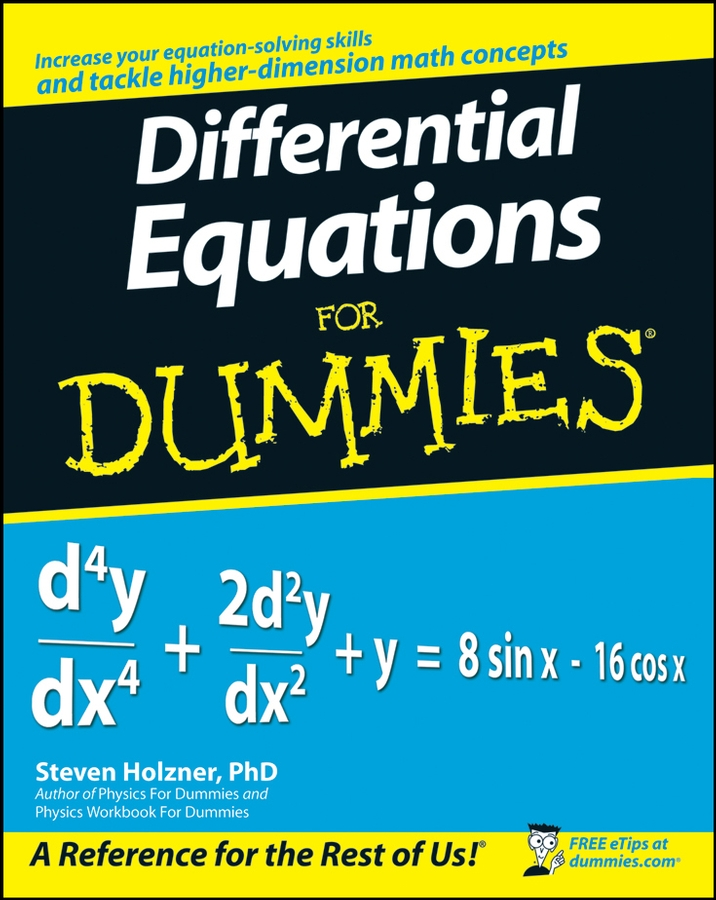A quadratic equation is any second-degree polynomial equation — that’s when the highest power of x, or whatever other variable is used, is 2. The solution or solutions of a quadratic equation,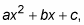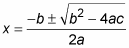Solve the equation,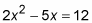1. Bring all terms to one side of the equation, leaving a zero on the other side.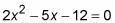2. Plug the coefficients into the formula.

In this example, a equals 2, b is –5, and c is –12, so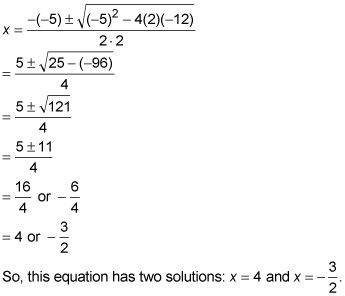You can also use the quadratic formula for factoring trinomials.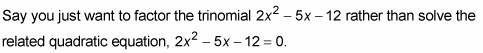Here’s what you do.

1. Use the quadratic formula to get solutions for x. (You can also use your calculator to get the solutions.) Make sure the solutions are written as fractions rather than as decimals and that they’re reduced to lowest terms.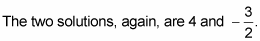2. Take the two solutions and put them in factors. If a solution is positive, use subtraction. If a solution is negative, use addition.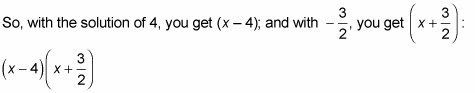3. If either solution is a fraction, take the denominator and bring it in front of the x.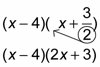And, voilà, the trinomial is factored:

(x – 4)(2x + 3)